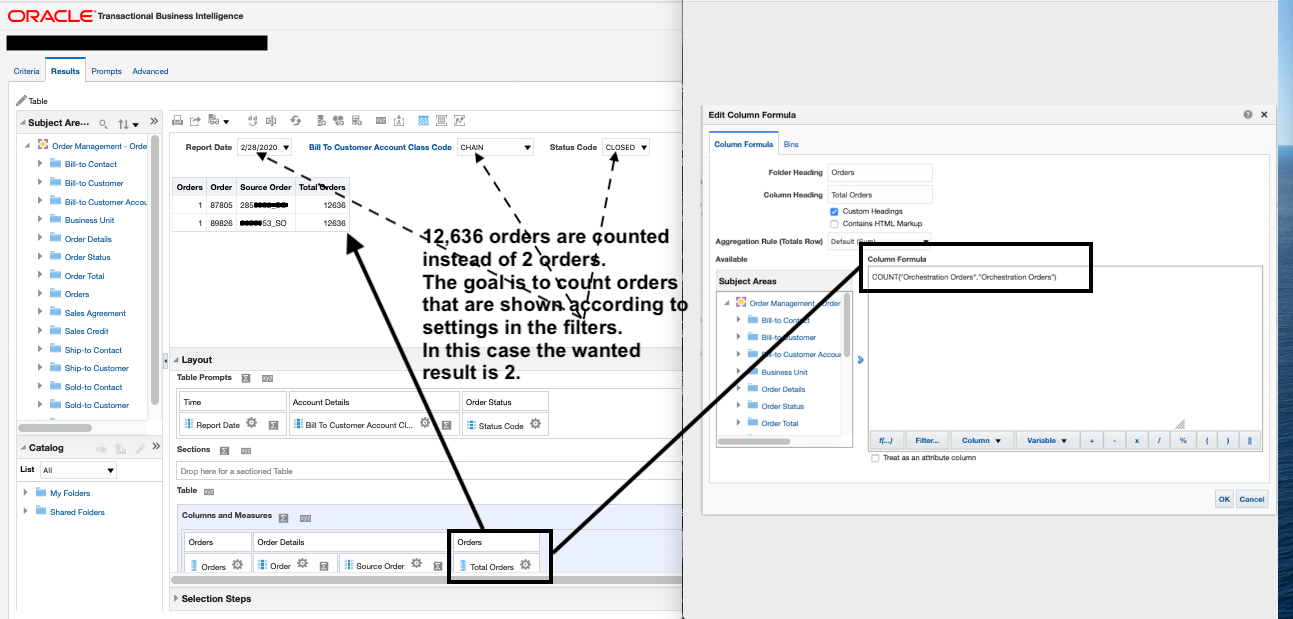67
Views
2

# Summary

How to only count the number of rows showing in an analysis

# Content

Hello Oracle communities,

When I attempt to display the total number of rows shown in an analysis in BI, and it resulted in the number of rows counted (12636) being beyond the number of rows displayed (2) per enclosed screenshot.  This over counting happens with both count() and sum() functions.  The "Orders" column, data be counted, is a fact component.

I also tried running count() on the "Order" column, a dimension column showing the number ID of an order.  "1" showed up per each row under that new calculated column.

What should be changed to the formula so it produces a total number of orders that match with the number of orders shown in the analysis?  In this case 2, instead of 12636.

Thank you.

# VersionTagged: